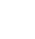# Tag Archives: get column letter

## Get the current cells column letter

A formula to return the column letter(s) when placed in any cell. For instance if the formula was put in cell G56 the result in cell G56 would be G. If it was put in IMB213 then the result would be IMB. =LEFT(SUBSTITUTE(ADDRESS(ROW(),COLUMN()),”\$”,””),LEN(SUBSTITUTE(ADDRESS(ROW(),COLUMN()),”\$”,””))-LEN(ROW()))